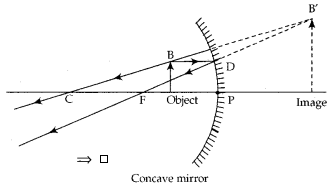# (a) To construct a ray diagram we use two rays which are so chosen that it is easy to know

(a) To construct a ray diagram we use two rays which are so chosen that it is easy to know their directions after reflection from the mirror. List two such rays and state the path of these rays after reflection in case of concave mirrors. Use these two rays and draw ray diagram to locate the image of an object placed between pole and focus of a concave mirror.

(b) A concave mirror produces three times magnified image on a screen. If the object is placed 20 cm in front of the mirror, how far is the screen from the object?

(a) These two rays are:
(i) Ray of light parallel to principal axis, passes through point F of concave mirror after its reflection.
(ii) Ray of light passing through point C of the mirror, is reflected back on the same path after reflection from the mirror.(b)Distance between the screen and the object distance = [-60 - (-20)] = -40 cm
So, the screen is at a distance of 40 cm from the object. Minus sign is only as per sign conventions.